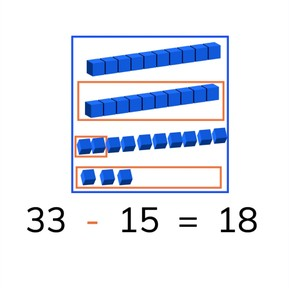Subtraction to 50 with subtrahends >10

# Subtraction to 50 with subtrahends >10

I can subtract numbers greater than 10 from numbers to 50.

No account needed.8,000 schools use Gynzy92,000 teachers use Gynzy1,600,000 students use Gynzy

## General

Students learn to subtract with numbers greater than ten up to 50. They also cross tens.

2.NBT.B.5

## Relevance

It is important that students are able to subtract numbers to 50 so that they can determine how many they have left with larger quantities.

## Introduction

Start with a subtraction problem in which students are asked to drag blocks into a box to show subtraction. Then they practice a subtraction problem to 30 on the number line with a minuend greater than ten.

## Development

Discuss the importance of subtraction to 50. This learning goal will be taught in three forms, visual, abstract and in story form. You may use the blue menu in the bottom right to select which form is best suited to your classroom needs. Each form starts with explanation, then practicing together as a class, and ends with students practicing on their own or in small groups to check their learning. The first form is visual. Explain a subtraction problem by using the blocks on the interactive whiteboard. Do a problem as a class and then ask students to solve the next visual problem. Next the abstract problems are given. Tell students that one way of subtracting is to first subtract the tens, then count back to the next ten, and then take away the rest. It is helpful to imagine a number line while doing this. You can also decompose both numbers and subtract the tens and then subtract the ones. Practice a few examples and then have students do a few problems on their own. Finally go through the steps of solving a story problem with the class and solve the given story problem. Ask students if they can solve the next story problem in pairs, and the final story problem individually.

To check that students understand subtraction to 50 with minuends greater than ten you can ask the following questions:
- Why is it useful to be able to subtract numbers to 50?
- How do you subtract numbers that cross tens? For example 45-17.

## Guided practice

Students are given subtraction problems in the visual, abstract and story problem forms.

## Closing

Ask students to do one subtraction problem in each form, visual, abstract, and story problem. Then divide your class into groups. Each group should get 3 six-sided dice, or 2 10-sided dice. Each player starts with 50 points. Throw the dice. Those numbers must be subtracted from their 50 points. Who reaches 0 first? If you subtract to "more" than zero, you must throw the dice again.

## Teaching tips

Students who have difficulty with this can be supported with the use of manipulatives or use of a number line.

## Instruction materials

3 six-sided dice or 2 ten-sided dice per group
Optional: blocks or other manipulatives

### The online teaching platform for interactive whiteboards and displays in schools

• Save time building lessons

• Manage the classroom more efficiently

• Increase student engagement